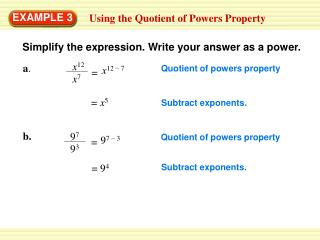DownloadDownload PresentationEXAMPLE 3

# EXAMPLE 3

Download Presentation## EXAMPLE 3

- - - - - - - - - - - - - - - - - - - - - - - - - - - E N D - - - - - - - - - - - - - - - - - - - - - - - - - - -
##### Presentation Transcript

1. x12 a. x12 – 7 = x7 97 93 b. 97 – 3 = EXAMPLE 3 Using the Quotient of Powers Property Simplify the expression. Write your answer as a power. Quotient of powers property = x5 Subtract exponents. Quotient of powers property = 94 Subtract exponents.

2. y4y a. = y3 b. xy4 – 3 = xy4 y5 y3 y3 EXAMPLE 4 Simplifying Fractions with Powers Simplify the expression. Simplify numerator using product of powers property. = y5 – 3 Quotient of powers property. = y2 Subtract exponents. Quotient of powers property. = xy Subtract exponents.

3. a6 9. a4 109 106 10. for Examples 3 and 4 GUIDED PRACTICE Simplify the expression. Write your answer as a power. = a2 = 103

4. q3 q5 11. q4 for Examples 3 and 4 GUIDED PRACTICE Simplify the expression. Write your answer as a power. = q4

5. a2b8 12. b2 for Examples 3 and 4 GUIDED PRACTICE Simplify the expression. Write your answer as a power. = a2b6